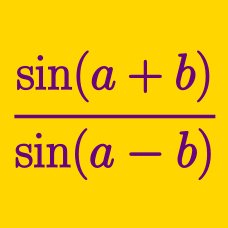Geometry

# Product to Sum Trigonometric Formulas

If $a \neq 0, \sin(x+y) = \frac{11}{a}, \sin(x-y) = \frac{2}{a},$ what is $\displaystyle{\frac{\tan x}{\tan y}}?$

If $\cos \alpha=\frac{1}{11}$ and $\cos \beta=\frac{10}{11}$, the value of $\sin (\alpha+\beta) \sin (\alpha-\beta)$ can be expressed as $\frac{a}{b}$, where $a$ and $b$ are coprime positive integers. What is the value of $a+b$?

$N$ is an integer in the range $[0, 180]$ such that

$2 \cos 43 ^\circ \cos 26 ^\circ = \cos 69 ^ \circ + \cos N ^ \circ.$

What is the value of $N$?

If $a \neq 0, \sin(x+y) = \frac{12}{a}, \sin(x-y) = \frac{5}{a},$ what is $\displaystyle{\frac{\tan x}{\tan y}}?$

Which of the following is equal to $\cos 6 \theta \cos 2 \theta?$

×